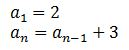# Using Recursive Rules for Arithmetic, Algebraic & Geometric Sequences - Quiz & Worksheet

Instructions:

question 1 of 3

### Which of the sequences below has the recursive rule where each number is the previous number times 2?

Create Your Account To Take This Quiz

As a member, you'll also get unlimited access to over 84,000 lessons in math, English, science, history, and more. Plus, get practice tests, quizzes, and personalized coaching to help you succeed.

Try it risk-free for 30 days. Cancel anytime

### 1. What is the recursive rule here?### 2. Which of the following sequences follows this formula.Create your account to access this entire worksheet
Quizzes, practice exams & worksheets
Certificate of Completion
Create an account to get started

Recursive rules can be used to take one number and turn it into another. This quiz/worksheet combo will test your ability to use and identify recursive rules in practice.

## Quiz & Worksheet Goals

In these assessments, you'll be tested on:

• Figuring out what sequence matches a recursive rule
• Identifying a recursive rule
• Matching a sequence with a formula
• Matching a rule with a formula

## Skills Practiced

This quiz and worksheet allow students to test the following skills:

• Reading comprehension - ensure that you draw the most important information from the relate lesson on recursive rules
• Problem solving - use acquired knowledge to solve recursive rule practice problems
• Information recall - access the knowledge you've gained regarding recursive rules

To learn more about recursive rules, review the accompanying lesson titled Using Recursive Rules for Arithmetic, Algebraic & Geometric Sequences. This lesson covers the following objectives:

• Define recursive rules
• Demonstrate how a recursive rule works
• Show how to use a recursive formula
• Provide examples and practice problems for recursive formulas
Final ExamAlgebra II Textbook
Status: Not Started
Chapter ExamAlgebra II: Sequences and Series
Status: Not Started

Support